# Integration

Integration

In this section, we will explore the world of integration. We will do so by learning the examples on integration, integration rule, and other integral concepts. You can check out the interactive simulations to know more about the lesson and try your hand at solving a few interesting practice questions at the end of the page.

Let's recap a bit before diving into integration. You already have an intuitive idea of derivatives.

If we differentiate a function $$f$$ in an interval $$I$$, then we get a family of functions in $$I$$.

What if the values of functions are known in $$I$$?

Can you determine the function $$f$$?

Yes, we do it using the method of integration.

Basically, integration is a way of uniting the part to find a whole.

A pizza is integration and each slice of the pizza is the derivative.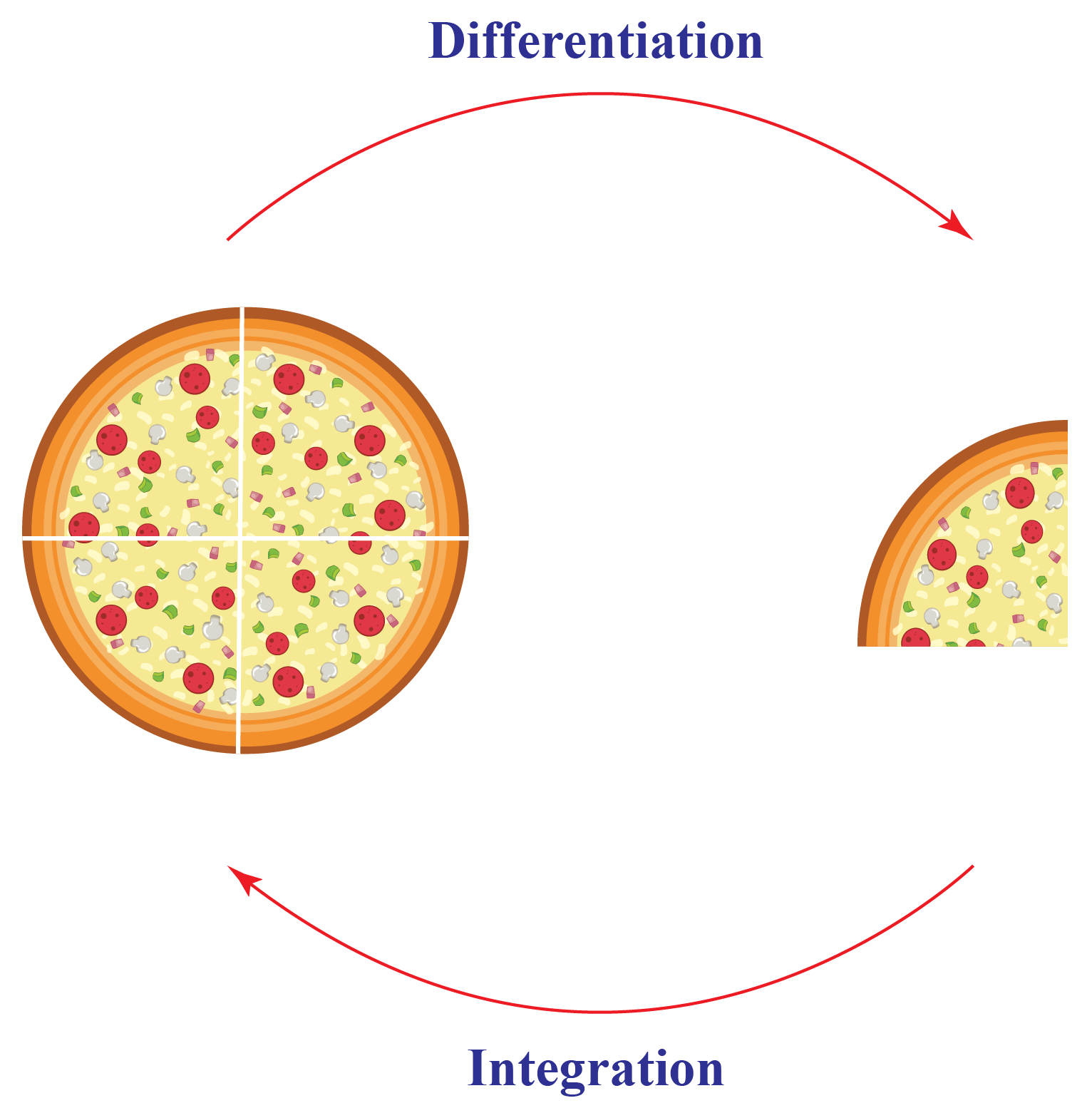Here, the heart of the matter is the integration of a function in its domain of definition.

Let's move further and learn about integration and some of its powerful techniques.

## Lesson Plan

 1 What Is Meant By Integration? 2 Important Notes on Integration 3 Solved Examples on Integration 4 Challenging Questions on Integration 5 Interactive Questions on Integration

## What Is Meant By Integration?

Given the derivative $$f’$$ of the function $$f$$, the natural question arises is can we determine the function$$f$$?

The functions that give the derivative, that is, the function $$f$$ is called antiderivative or integral of $$f’$$.

The process of finding the antiderivative is called integration.

For example, the derivative of $$f(x)=x^{3}$$ is $$f’(x)=3x^{2}$$ and the antiderivative of $$g(x)=3x^{2}$$ is $$f(x)=x^{3}$$.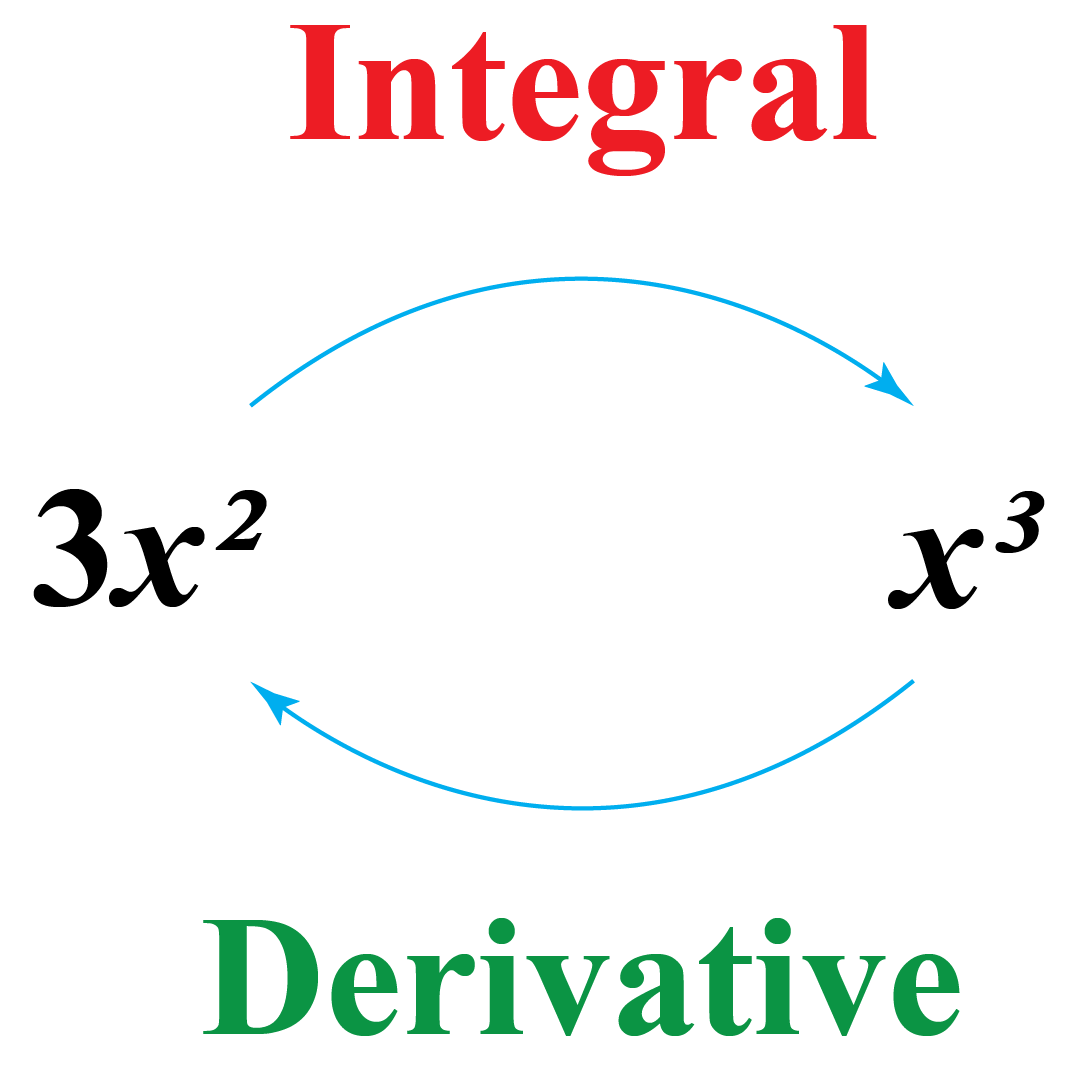## How Integration Is an Inverse Process of Differentiation?

By definition of integration, we know that integration is an inverse process of differentiation.

If we are given the derivative of a function, the process of finding the original function is called integration.

Consider a function $$f(x)=\dfrac{x^{3}}{3}$$.

We know that, $$\dfrac{d}{dx}\left(\dfrac{x^{3}}{3}\right)=x^{2}\;\;\;\;\;\; \cdots (1)$$

Here, we say $$\dfrac{x^{3}}{3}$$ is the integral of $$x^{2}$$.

In fact, there exists infinite integrals of this function because the derivative of any real constant $$C$$ is zero and we can write (1) as $$\dfrac{d}{dx}\left(\dfrac{x^{3}}{3}+C\right)=x^{2}$$

So, it is an integration rule to add an arbitrary constant $$C$$ from the set of real numbers.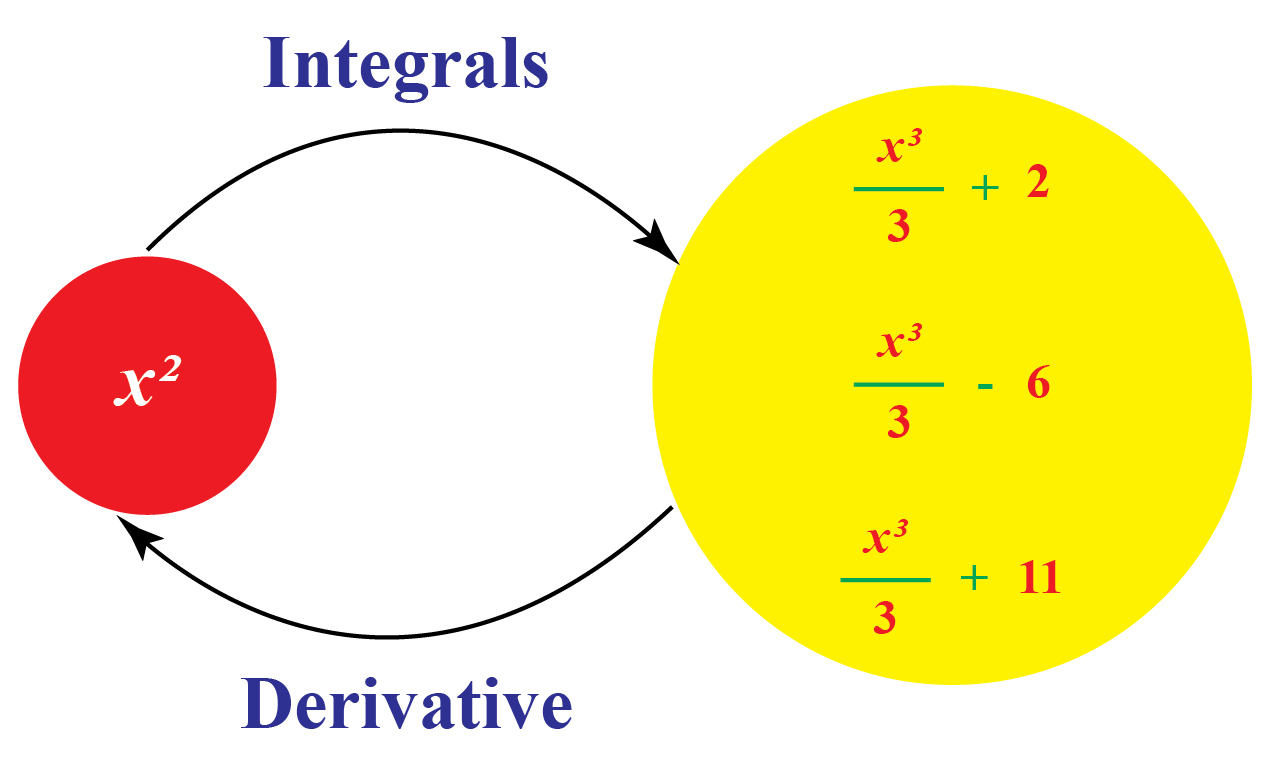### Notation

If $$\dfrac{dy}{dx}=f(x)$$, we write $$y=\int f(x) dx$$.

Here, $$\int f(x) dx$$ represents the whole class of integral.

This is read as "Integral of $$f$$ with respect to $$x$$".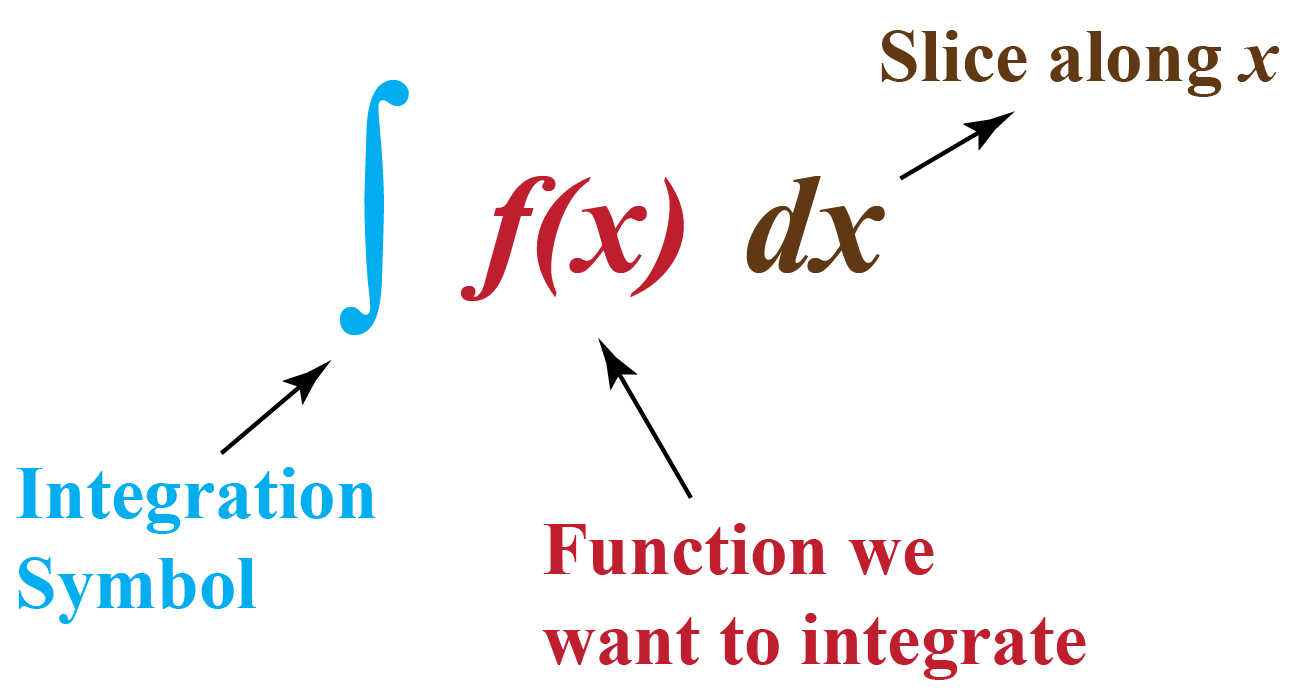## Formulas of Integration

We already know formulas of derivatives of some important functions.

Here are the corresponding integrals of these functions.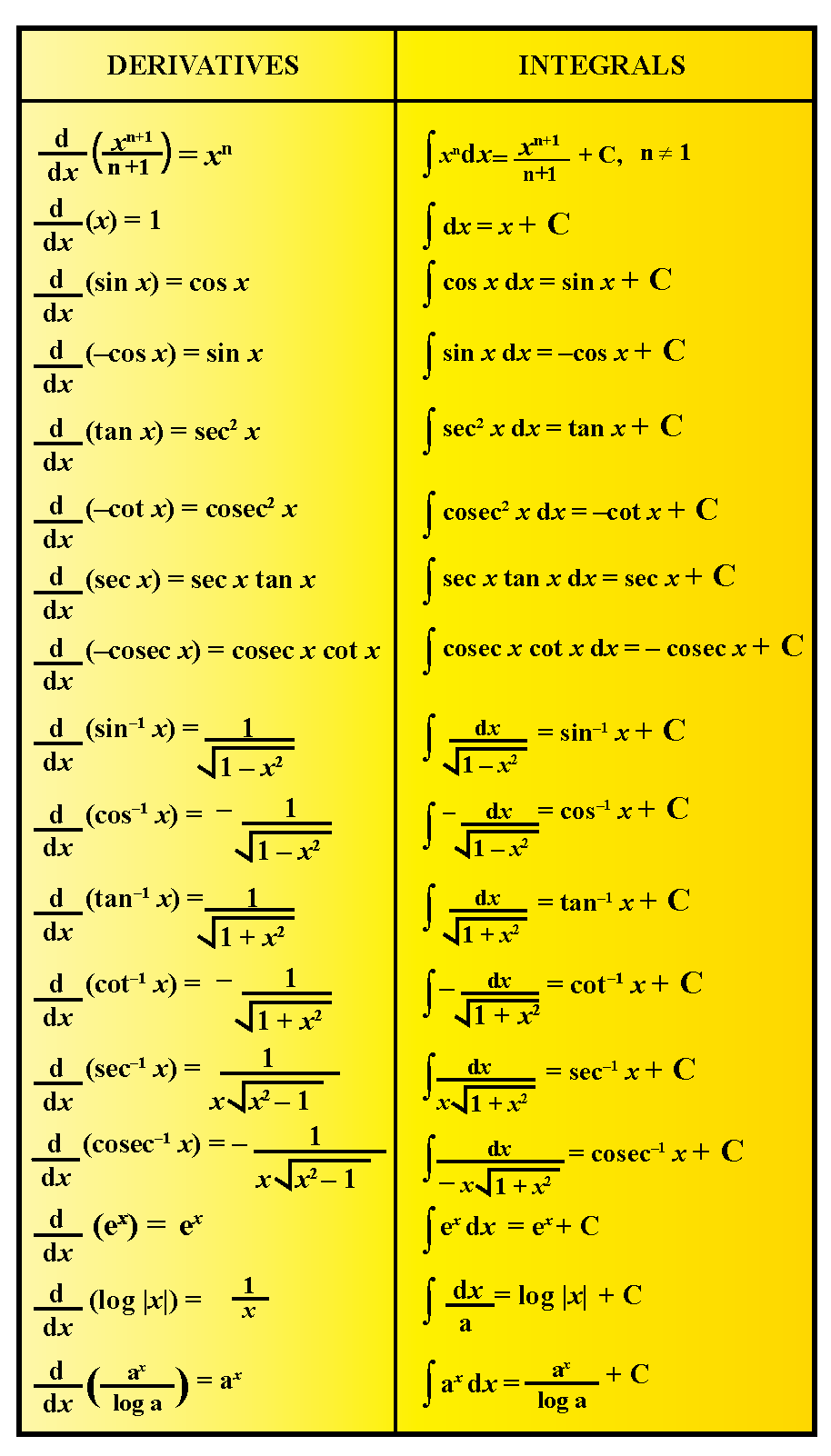## What Are the Different Methods of Integration?

Sometimes, the inspection is not enough to find the integral of some functions.

There are additional methods to reduce the function in the standard form to find its integral.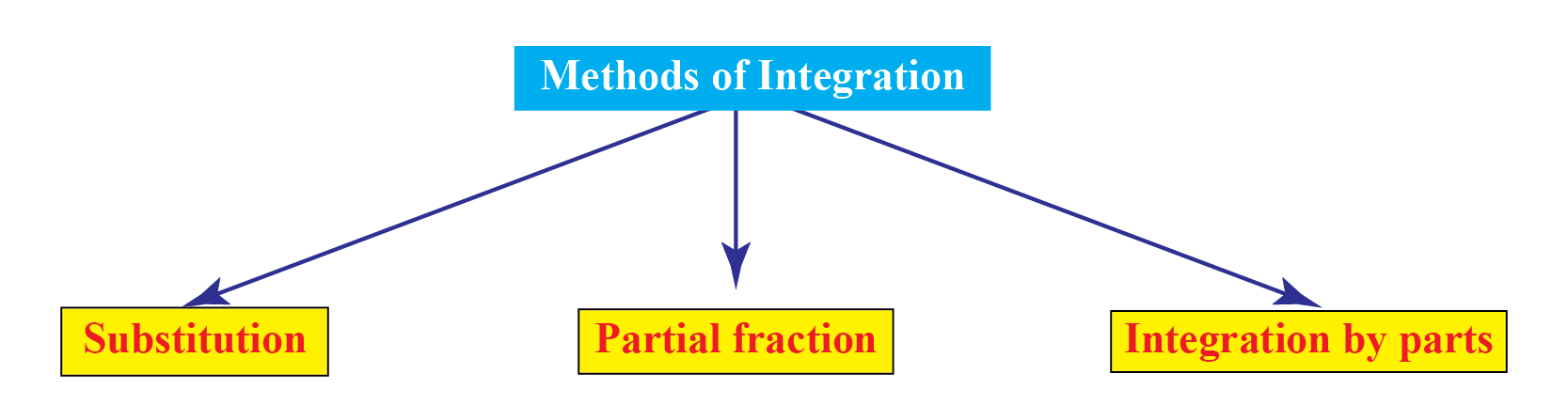Prominent methods are given below.

### Method 1: Substitution

Suppose, we have to find $$y=\int f(x) dx$$.

Let $$x=g(t)$$. Then, $$\dfrac{dx}{dt}=g'(t)$$.

So, $$y=\int f(x) dx$$ can be written as $$y=\int f(g(t)) g'(t)dt$$.

For example, let's find the integral of $$f(x)=\sin{mx}$$ using substitution.

Let $$mx=t$$. Then, $$m\dfrac{dx}{dt}=1$$.

So,

\begin{align}y&=\int \sin{mx}dx\\&=\dfrac{1}{m}\int \sin{t}dt\\&=-\dfrac{1}{m} \cos{t}+C\\&=-\dfrac{1}{m} \cos{mx}+C\end{align}

$$y=\int \sin{mx}dx$$ can be written as $$y=\int f(g(t)) g'(t)dt$$.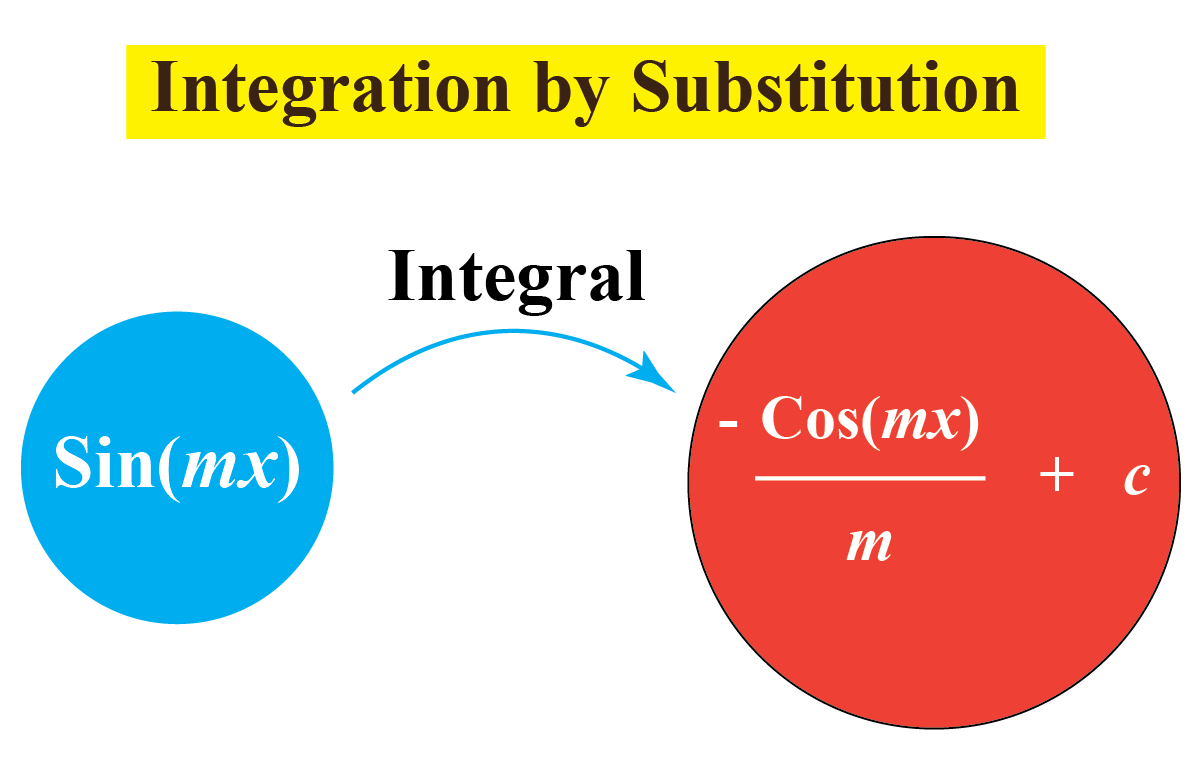Here is a simulation for you to practice integration by substitution.

### Method 2: Partial Fractions

Suppose we have to find $$y=\int \dfrac{P(x)}{Q(x)} dx$$ where $$\dfrac{P(x)}{Q(x)}$$ is an improper rational function.

We reduce it in such a way that $$\dfrac{P(x)}{Q(x)}=T(x)+\dfrac{P_{1}(x)}{Q(x)}$$.

Here, $$T(x)$$ is polynomial in $$x$$ and $$\dfrac{P_{1}(x)}{Q(x)}$$ is proper rational function.

The following table shows some rational function and their corresponding rational functions.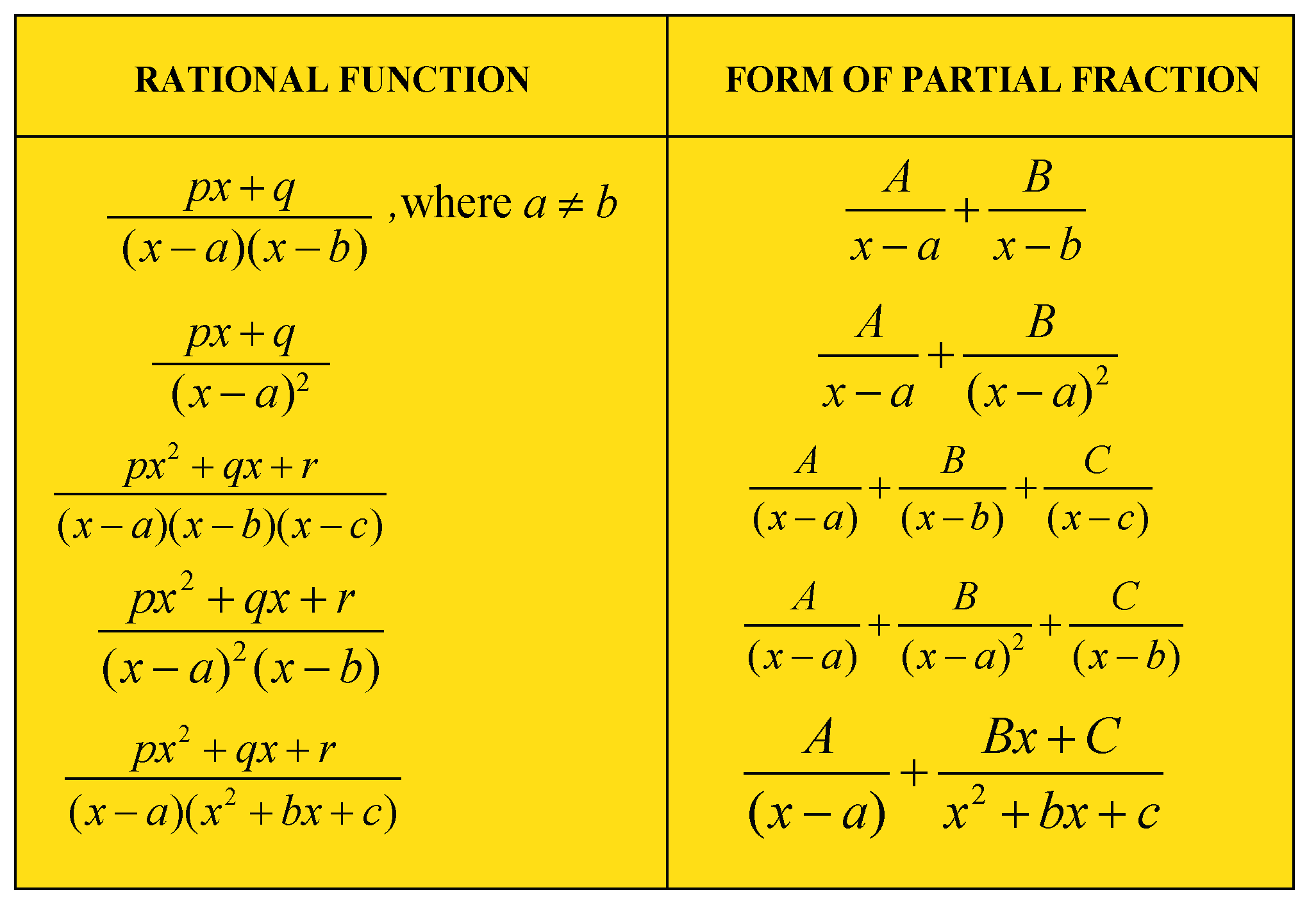For example, let's find the integral of $$f(x)=\dfrac{1}{(x+1)(x+2)}$$ using partial fraction.

By using partial fraction we have $$\dfrac{1}{(x+1)(x+2)}=\dfrac{A}{x+1}+\dfrac{B}{x+2} \cdots (1)$$.

We will determine the values of $$A$$ and $$B$$.

On comparing in equation (1), we get $$1=A(x+2)+B(x+1)$$.

From this, we have a set of two linear equations.

\begin{align}A+B&=0\\2A+B&=1\end{align}

On solving these equations we get, $$A=1$$ and $$B=-1$$.

So, equation (1) can be written as $$\dfrac{1}{(x+1)(x+2)}=\dfrac{1}{x+1}-\dfrac{1}{x+2}$$.

Now, solving the integral

\begin{align}\int \left(\dfrac{1}{(x+1)(x+2)}\right)dx\\=\int \left(\dfrac{1}{x+1}-\dfrac{1}{x+2}\right)dx\\=\log{|x+1|}-\log{|x+2|}+C\\=\log{\left|\dfrac{x+1}{x+2}\right|}+C\end{align}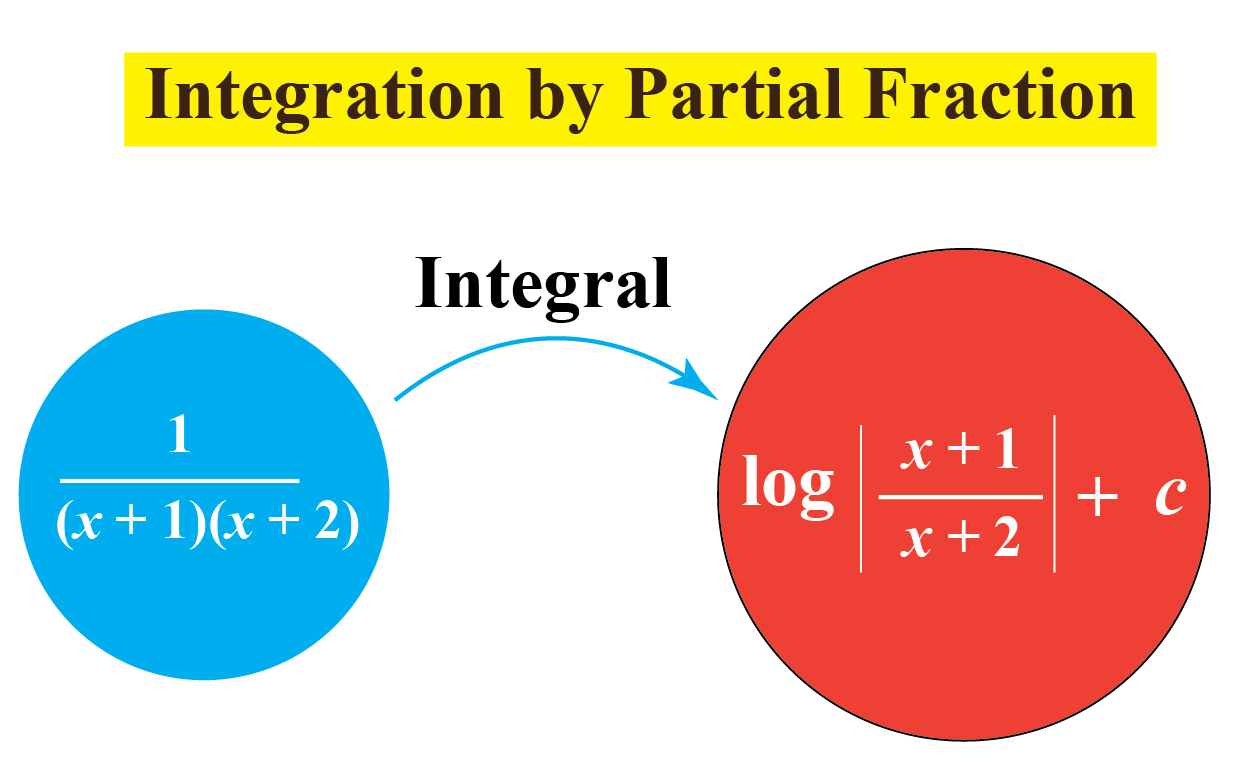Experiment with the simulation below to practice this method.

### Method 3: Integration by Parts

This Integration rule is used to find the integral of two functions.

By product rule of derivatives, we have $$\dfrac{d}{dx}(uv)=u\dfrac{dv}{dx}+v\dfrac{du}{dx}\;\;\;\;\;\;\; \cdots (1)$$

Integration on both sides of equation (1), we get $$\int u\dfrac{dv}{dx}dx=uv-\int v\dfrac{du}{dx} dx\;\;\;\;\;\;\; \cdots (2)$$

Equation (2) can be written as $$uv=\int u\dfrac{dv}{dx}dx+\int v\dfrac{du}{dx} dx$$

Let $$u=f(x)$$ and $$\dfrac{dv}{dx}=g(x)$$.

Then, we have $$\dfrac{du}{dx}=f'(x)$$ and $$v=\int g(x)dx$$.

So, Equation (2) becomes \begin{align}\int f(x) g(x)dx\\=f(x) \int g(x)dx-\int [f'(x) \int g(x)dx]dx\end{align}

For example, let's find the integral of $$xe^{x}$$ using integration by parts.

\begin{align}\int xe^{x}dx&=x \int e^{x}dx-\int \left[\dfrac{dx}{dx}\int e^{x}dx\right]dx\\&=x e^{x}-\int [e^{x}]dx\\&=x e^{x}-e^{x}+C\end{align}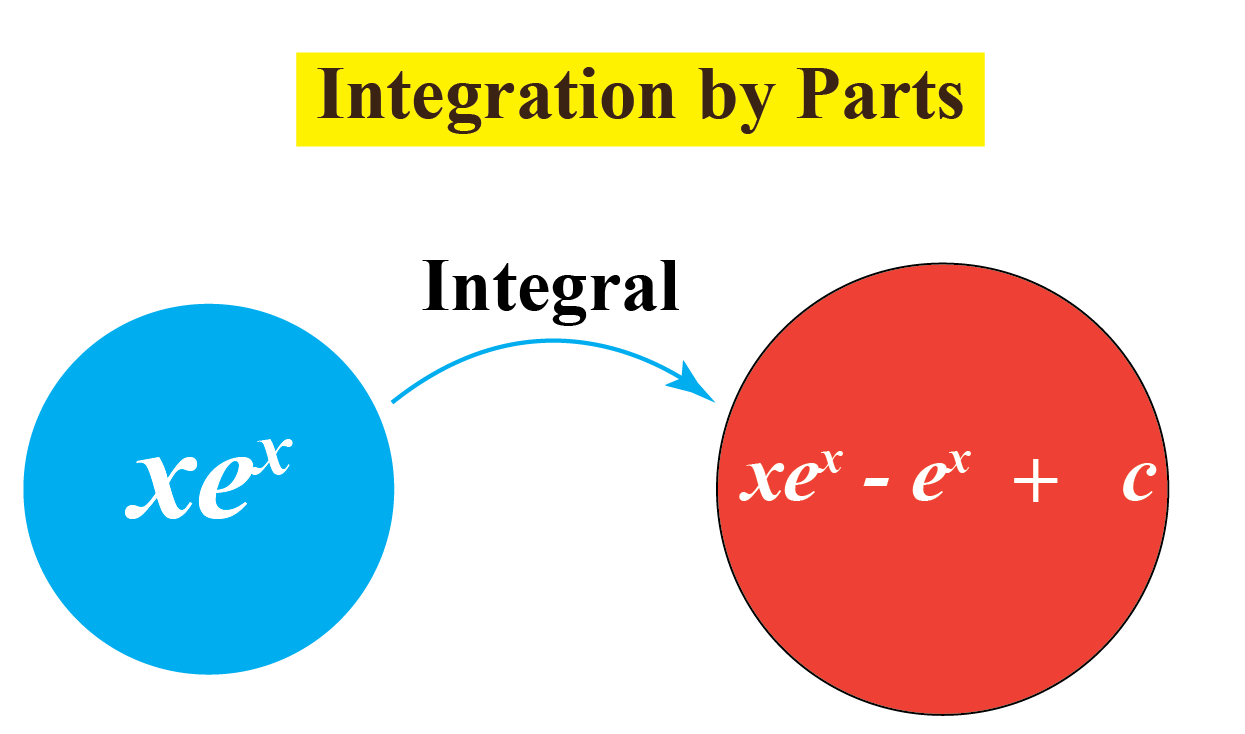Try this method in the simulation below.

## Let's Summarize

We hope you enjoyed learning about Integration with the examples and practice questions. Now, you will be able to easily solve problems on integration, examples on integration, integration rule, and integrals.

## About Cuemath

At Cuemath, our team of math experts is dedicated to making learning fun for our favorite readers, the students!

Through an interactive and engaging learning-teaching-learning approach, the teachers explore all angles of a topic.

Be it worksheets, online classes, doubt sessions, or any other form of relation, it’s the logical thinking and smart learning approach that we, at Cuemath, believe in.

## 1. What is the integration of 1?

The integration of 1 is $$x+C$$

## 2. How integration is used in real life?

The applications of integration in real-life is mentioned below.

1. In electrical engineering, we need a cable to connect two substations that are miles apart from each other. Integration helps us to find the exact length of the cable.
2. In physics, integration is used to find the center of mass, the center of gravity, the velocity of an object, etc.
3. In epidemiology, integral calculus is used to study the spread of an infectious disease.

## 3. Why is the integration important?

Calculus is centered on the concepts of derivatives and integration.

In mathematics, we use integration to find the areas, volumes, displacement, etc.

In fact, the concept of integration in calculus gave birth to integral calculus.

More Important Topics
Numbers
Algebra
Geometry
Measurement
Money
Data
Trigonometry
Calculus